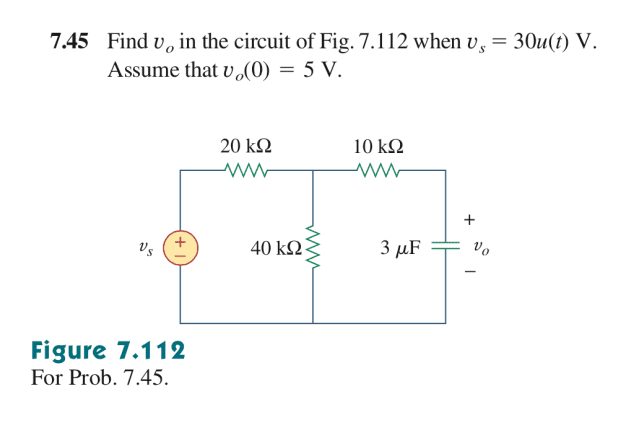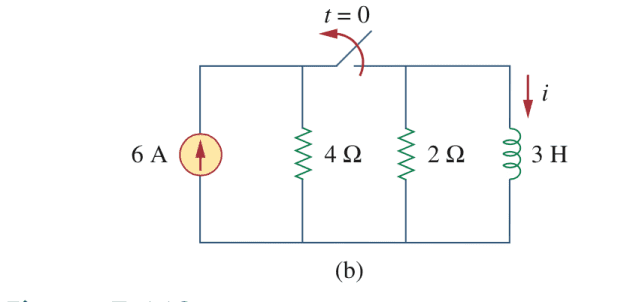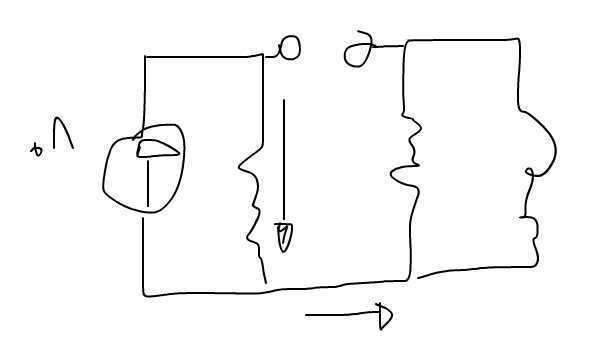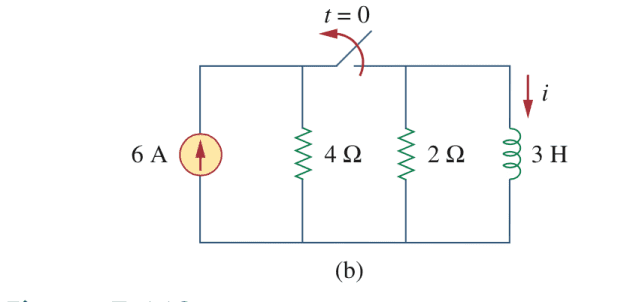# RC circuits, a step skipped in solution

• Engineering

## Homework Statement

.
[/B]This is problem 7.45 in Fundamental of Electric Circuit 5th edition.
2. Homework Equations

First off, I want to go ahead and say that I don't need the solution I already solved it.

I went ahead and used Nodal analysis when Vs = 30. I placed a node voltage right above the 40kΩ wire say ##V_1##.
I then solved for V1 using Voltage division,
##V_1 = \frac{40} {40+20} * 30 = 20## .

Applying nodal analysis,

##\frac{V_1-30}{20} + \frac{V_1}{40} + \frac{V_1-V_o}{10} = 0##.

∴ ##V_o = 20V##

To my understanding Vo is not equal to V1 because there's a resistor between them. However, the problem is that in the textbook solution assumed it WAS and skipped the nodal analysis step.

After solving my nodal equation I realize indeed that Vo is actually equal to V1.
Could anyone explain why? I'd like to know this so I could stop myself from using Nodal analysis for no reason in the final exam.

Please have mercy on me this is my first post

Last edited:

gneill
Mentor
Hi BarelyOtaku, Welcome to Physics Forums!

Note that resistors alone do not cause potential drops. It's current flowing through a resistor that causes a potential drop.

In your circuit, once steady state has been achieved "after a long time", the capacitor has reached some constant voltage and charge and current to or from the capacitor is zero. That makes the current through the 10k resistor also zero. As a result there is no potential drop across that resistor: it must have the same potential at both of its ends.

•BarelyOtaku
Alright thank you very much! Sorry, you replied pretty fast so I didn't expect a reply.
That explanation makes sense but now I've come into the exact same problem but with current xDI couldn't grasp the concept of what happens after the switch is open. The book says it's a source-free RL circuit.
However, couldn't the current just flow through the 4Ω resistor and into the RL circuit?Last edited:
gneill
Mentor
Last edited by a moderator:
•BarelyOtaku
donpacino
Gold Member
Alright thank you very much! Sorry, you guys replied pretty fast so I didn't expect a reply.
That explanation makes sense but now I've come into the exact same problem but with current xDI couldn't grasp the concept of what happens after the switch is open. The book says it's a source-free RL circuit.
However, couldn't the current just flow through the 4Ω resistor and into the RL circuit?
View attachment 82283
current has to have a path to ground. Current would not have a path.

If you're confused, write a KCL or KVL at that node.

•BarelyOtaku
Oooooh, my mind just exploded from comprehending these basic steps.
I was never told and never understood these fundamentals of current and voltage, nor did I find a reference to understand them; now it makes a lot more sense now,
thank you so much guys!

donpacino
Gold Member
I just want to clarify, are you supposed to find the DC steady state value, or are you trying to find the step response of the systems?

Oh I'm supposed to find the inductor current equation for before and after the switch is turn on

NascentOxygen
Staff Emeritus
Oh I'm supposed to find the inductor current equation for before and after the switch is turn on
Careful. You are asked to calculate the current before and after the source is disconnected from the inductor, which means after the switch is opened.

Are you required to find the final steady-state current, or are you looking for current as an exponential function of time?

Well from my understanding before the switch is turned on would be the final steady-state, and after the switch is turned on would be the exponential function of time, since it decays as a source-free function.

Careful. You are asked to calculate the current before and after the source is disconnected from the inductor, which means after the switch is opened.

Are you required to find the final steady-state current, or are you looking for current as an exponential function of time?

Well from my understanding both.
Before the switch is turned on would be the final steady-state, and after the switch is turned on would be the exponential function of time; since it decays as a source-free function.

donpacino
Gold Member
So the answer you gave for both problems was only the steady state value of these circuits, after at least 5 time constants have passed

So the answer you gave for both problems was only the steady state value of these circuits, after at least 5 time constants have passed
Yeah I know, I didn't put the whole solution. I was only highlighting the part of the solution I was confused about.

NascentOxygen
Staff Emeritus
Before the switch is turned on would be the final steady-state,
I was trying to encourage you to use meaningful terms, terms that will be understood by others. If you get the conventions correct at this early stage in your studies, you'll be set for life. Get them wrong, and you'll find it difficult to unlearn later.

We do not see the switch here turn on. Turning a switch on means making a connection, and we are not witness to that happening here.

The switch has already been ON for a long time before t=0 and conditions have reached a steady state. Then at t=0 the source is switched OFF by moving the switch contacts to the position shown in the diagram, and thereafter some time elapses before the inductor branch reaches a new steady state.

There are two steady state conditions. Between the two, there is a period where transient conditions prevail---for the inductor best described as an exponential decay.

I was trying to encourage you to use meaningful terms, terms that will be understood by others. If you get the conventions correct at this early stage in your studies, you'll be set for life. Get them wrong, and you'll find it difficult to unlearn later.

We do not see the switch here turn on. Turning a switch on means making a connection, and we are not witness to that happening here.

The switch has already been ON for a long time before t=0 and conditions have reached a steady state. Then at t=0 the source is switched OFF by moving the switch contacts to the position shown in the diagram, and thereafter some time elapses before the inductor branch reaches a new steady state.

There are two steady state conditions. Between the two, there is a period where transient conditions prevail---for the inductor best described as an exponential decay.

I was just referring to the second problem 7.53 where there's a switch. When donpacino asked me the question I assumed he meant the second one since the first one clearly has it's objective defined. Unless I assumed wrong, but I'm pretty sure he was talking about the second one.

Thanks for the clarification, I appreciate as much help as I could get!

NascentOxygen
Staff Emeritus